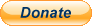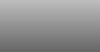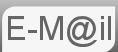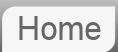# Answer to Riddle #77: Where's the Father

77. A mother is 21 years older than her child. In exactly 6 years from now, the mother will be exactly 5 times as old as the child.

Where's the father?

Just a quickie here. If you think about it the answer can only be one thing. There's only one time when we know where the father is and it will have to solve to that. Nice twist on a classic puzzle though:Before reading the answer can I interest you in a clue?

The technique to solving these age related puzzles, and there's a lot of them, is to convert the question in to two equations and solve them. Technically these are called simultaneous equations, with two equations and two unknowns. Though really it's just common sense.

Let's have M be the age of the mother & C the age of the child. In the time frame of now
'mother is 21 years older than her child'
M = C + 21

Make sure you get this the right way around, it can be a bit of a trap. 'mother is 21 years older than her child' means the child's age plus 21 is the mother's age, M = C + 21 not M + 21 = C

'exactly 6 years from now, the mother will be exactly 5 times as old as the child'
M + 6 = 5(C + 6)
M = 5C + 24

We now have two definitions of M in terms of C, we can put them together...

C + 21 = 5C + 24
21 = 4C + 24
C = -3/4

That is to say the child is minus ¾ years old, or minus 9 months. The time at which we know the father is with the mother!

Both AI's miss the trick here. Both pointing out that there is not enough information to know where the farther is.

If you're curious what Bard made of this puzzle...

If you're curious what ChatGPT made of this puzzle...

© Nigel Coldwell 2004 -  – The questions on this site may be reproduced without further permission, I do not claim copyright over them. The answers are mine and may not be reproduced without my expressed prior consent. Please inquire using the link at the top of the page. Secure version of this page.PayPal
I always think it's arrogant to add a donate button, but it has been requested. If I help you get a job though, you could buy me a pint! - nigel